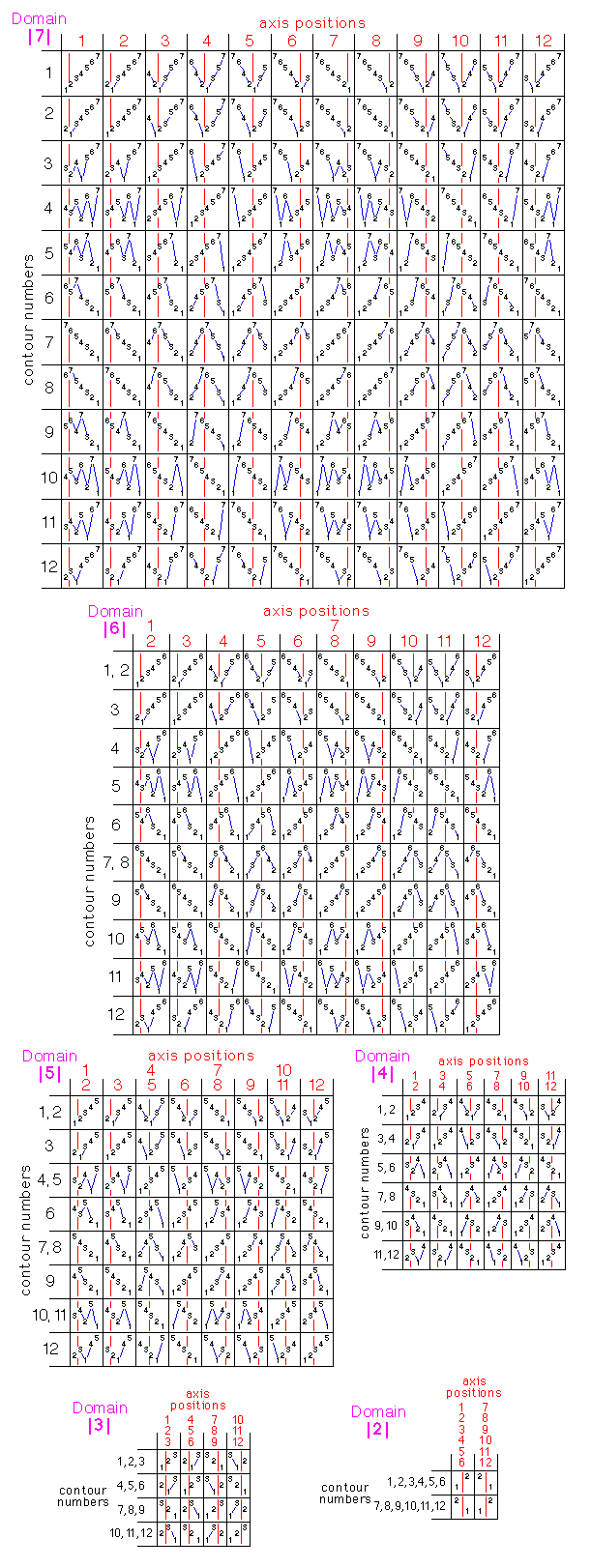﻿

Krystal Permutation

Introduction
Superstrands

Introduction

This document provides a detailed description of how Moritz’ permutation node permutes krystals. It assumes prior knowledge of the following documentation:

Permutation was not implemented in Krystals 4.0 (2005), even though it was part of Krystals 3.0 when I published my first website in 1999 (see, footnote 1 of the short introduction).
Krystals containing permuted values do however share some very useful — even indispensable — properties1, so I have now (December 2009) reprogrammed the original Krystals 3.0 pk function in Moritz’ permutation node: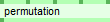This node creates a new krystal by permuting the values in its source krystal (the leftmost input krystal), using parameters set in the remaining input pins. The input pins are:
• Input pin 1 The source krystal
• Input pin 2 The axis input krystal
• Input pin 3 The contour input krystal
• Input pin 4 The permutation level
• Input pin 5 Sort first?

Superstrands

The easiest way to explain krystal permutation is first to define a new term: superstrand.
But before doing that, here is some recapitulation:

A strand is a sequence of one or more values having a level.
• A strand’s level is an integer which is greater than or equal to zero.
• Strand values are integers which are greater than 0.
In the rest of this document, example strands are represented with a red-coloured level, followed by a colon, followed by a list of comma-separated integers. For example:
3:7,3,5,7,12

Constant krystals have a single strand containing a single value at level 0.
For example ck0(17).krys contains the single strand
0:17
A single value cannot, of course, be permuted. So a constant krystal cannot be the source krystal for a permutation.

Non-constant krystals begin with a strand having level 1.
For example, the level 1 krystal lk1(7)-1.krys contains the strand
1:1,2,3,4,5,6,7
the values of which can be permuted.
The level 3 krystal xk3(1.7.2)-1.krys contains the strands
1:4,4,4,5
2:4,5,4,4
3:3,5,4,6,4
2:5,4,3,5
3:2,4,7,6,5
3:4,3,1
2:5,4,6,2
3:3,4,5,7,3,4
3:1,2,5,4,6
3:3,2
2:3,4,1,5,3,2,4
3:3,1,2
3:7,6,3,4
3:2
3:1,2,3,5,1
2:2,1,2,3,4,2
3:1,2
3:1,3,2,1
3:2,1,2
3:1,3,1,2,1,2,1
3:1,4,2,1,1
2:2
3:1,1,1,3,1,2
3:1,1
3:1,1,1,1
3:1,1,1
3:1,1,1,1,1,1,1
3:1,1,1,1,1
This is the strand information which is stored in krystal (.krys) files. If any of these strands has more than one value, those values can be permuted.

In Moritz’s Strands Browser and Krystal Browser, the level information is not displayed directly, but is used to structure the nesting level in the tree representation. This leaves the display less cluttered for other information.
Moritz’ display, with the corresponding strands, is as follows: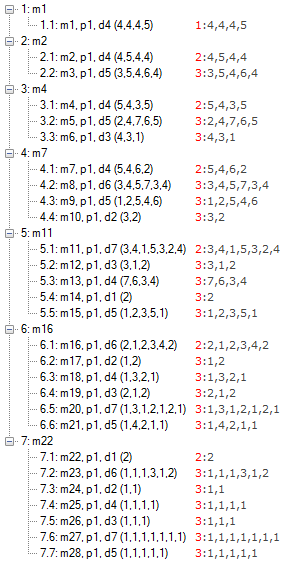Note that level of the first strand in each of the strand blocks is greater than zero, but less than the level of the lowest level strands (in this case 3), and that subsequent strands all have the krystal’s level (3).
Moritz can permute up to 7 elements at a time.2 The above krystal can be permuted at three levels, because all its density inputs (at all levels) had a maximum domain of 7. The three levels at which it can be permuted are:
• level 3: the (maximum 7) values in each strand
• level 2: the (maximum 7) strands in each of the above strand blocks
• level 1: the (maximum 7) the strand blocks themselves

These concepts can be generalized, leading to the following (recursive) definition:

Definition of a superstrand: A superstrand has a level, and contains either
1. a sequence of one or more superstrands whose level is numerically greater than the level of the containing superstrand, OR
2. a single strand.
Diagramatically:
A level 1 superstrand contains a list of
level 2 superstrands, each of which contains a list of
level 3 superstrands, each of which contains a list of
level 4 superstrands, each of which contains a list of
...
level x superstrands, each of which contains a single strand

The maximum superstrand level is equal to the maximum strand level in the krystal (which is the level of the krystal as a whole).

The above example krystal contains:
 1 level 1 superstrand containing 7 level 2 superstrands. 7 level 2 superstrands each of which contains up to 7 level 3 superstrands, 28 level 3 superstrands, each containing a single strand (each strand contains up to 7 values).

Note that
• All krystals contain a single, top-level superstrand. The top-level superstrand usually has level 1, but in constant krystals its level is 0.
• Strands are not superstrands (they dont “contain one or more superstrands or a single strand”).
• Superstrand levels are not strand levels: In the above example, a level 3 superstrand can contain strands of any level. The level 1 superstrand contains (at its strand level) strands of all levels.
• If the above (level 3) krystal were to be expanded to level 4, a new strand would be created for each of the values in the original krystal. The first of these would have the level of the original strand, and the rest would have level 4. Expansion converts the original strands to superstrands whose level is the level of the krystal which has been expanded (one less than the level of the resulting krystal).

Permutation

Moritz uses the standard contours from Krystals 3.03 to do permuting. An axis position and a contour number are used to retrieve one of the contours, and the permuted elements (superstrands or strand values) are sorted into the order given by the retrieved contour.

Unlike pk in Krystals 3.0, Moritz’ permutation node now has a boolean sort first parameter:
If sort first is false, the permutation is relative to the original positions of the elements:
Applying the contour 5647321 to elements 5334672 gives 6742335.
If sort first is true, the values are first sorted into “ascending order” before applying the permutation.
The original elements (e.g. 5334672) are sorted into ascending order (to 2334567), before applying the contour. Applying the contour 5647321 to 2334567, gives 5647332.
For strand values, sorting into "ascending order" simply means sorting according to the size of the integer values.
For superstrands, sorting into "ascending order" also means sorting according to size. Moritz defines the size of a superstrand as the total number of strand values it encompasses.

Permuting the contents of a superstrand should not change the size or level of any superstrand in the krystal, so, after doing the permutation, the levels of the first strands in each superstrand which has moved are restored to their original configuration.
For example, when permuting the content of the level 1 superstrand in the above krystal example, the blocks are first permuted (here with sort first set to false and contour 4352617) as in column 2, then the levels are restored as in column 3: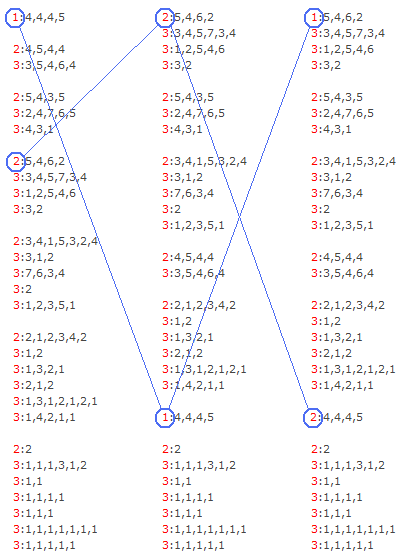Permuting the content of the level 2 superstrands (with sort first set to false, and axis=constant 1 and contour=constant 7 — the order of the level 3 superstrands is always reversed within each level 2 superstrand):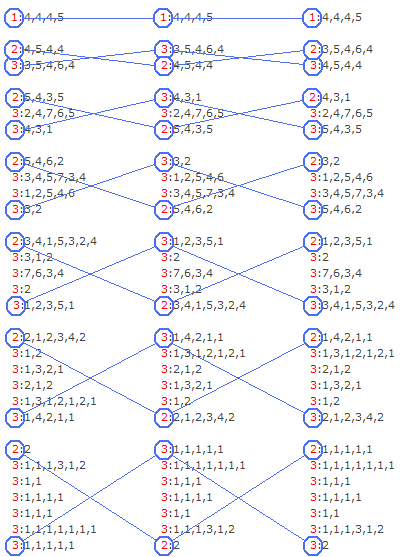Strand levels do not have to be restored when the values inside strands are permuted.

The axis and contour inputs

As in Moritz’ shaped expansion node, the axis and contour input krystals have a maximum domain of 12, so that their values can be used to retrieve standard contours. They must also share the density structure of the source krystal (the krystal which is being permuted) at their own level(s). (The axis and contour inputs do not have to have the same level.)
In the shaped expansion node, the levels of the axis and contour inputs simply have to be less than the level of the source krystal. (Permutation in that node is equivalent to using the permutation node being described here, at the level of the source krystal, with the sort first parameter set to true.)
Here, in the permutation node, the levels of the axis and contour input krystals must be less than the level of the permutation. For example, if the permutation level is set to 1 (the top permutable level), then the axis and contour inputs must have level 0 (constant krystals). If a level 6 krystal is being permuted at level 5, the axis and contour input krystals must have levels les than 5.
As an example of how lower level axis and contour inputs are expanded when their level is less than maximum, consider the following Moritz patch.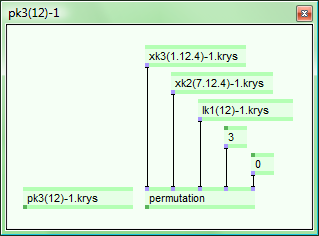Source (input): xk3(1.12.4)-1.krys. This krystal is being permuted at level 3 (its strand level).
Axis (input): xk2(7.12.4)-1.krys. This krystal has level 2, domain 12. Level 2 is the maximum level for the axis input, providing one value per strand in the output (see below).
Contour (input): lk1(12)-1.krys. Each of these values at level 1 is repeated to fill the shape of level 2. The resulting contour input values per strand can be seen in pk3(12)-1.krys’ ‘c’ column in diagram below. If the contour input had been a constant, the ‘c’ value would be the same during the whole permutation.
Level (input): 3
Sort First (input): 0 means false.
Output: pk3(12)-1.krys
The following diagram uses screenshots taken from the Krystals Browser’s display of the krystals’ content.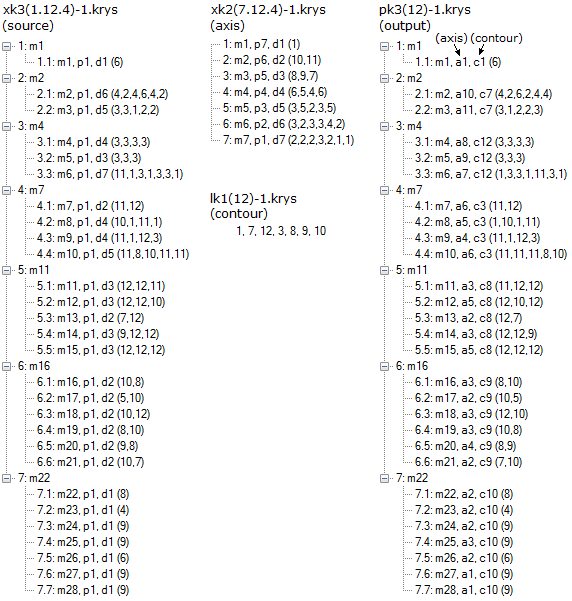Summary of the restrictions on the inputs to Moritz’ permutation node

Levels:
• The source krystal must have a level greater than 0, otherwise the level is unrestricted.
• The level which is being permuted must be greater than zero, and less than or equal to the level of the source krystal.
• The axis and contour levels do not have to be the same.
• The axis and contour levels must be less than the level which is being permuted.
Domains:
• There are no restrictions on the domain of the source krystal.
• The axis and contour domains must be less than or equal to 12. They do not have to be the same.
Density structure:
• The density input krystals which are ancestors of the source krystal should all have domains which are less than or equal to 7.
• The axis and contour input krystals (which do not have to be the same size) should have the same shape as one of the source krystal’s density ancestors.

The above restrictions are all implemented in the code of the permutation node. The node only opens pins for plausible potential inputs and, if this is not sufficient, an explanatory error message is presented as soon as possible.

Footnotes

1. Useful properties include:
• The total sum of the strand values remains constant under permutation. This can be useful when making bars “add up” (in space) when writing polyphonic music. The same is true, if strand values are associated with (i.e. mean) symbols having a particular logical width.
• The density of each level of superstrands remains the same when a krystal is permuted. This means that permuted krystals can be used interchangeably as arguments to the expansion node. For example, if xk3 is decended from xk2, and pk2 is a permutation of xk2, then if xk3 is the density input for an expansion, pk2 can be used as the points input. The values in pk2 are simply treated as level 2 values which are repeated according to the shape of xk3.
2. Restricting the maximum domain of a permutation to 7 was a conscious descision, having to do with ideas about cognitive psychology...
3. The standard contours are: